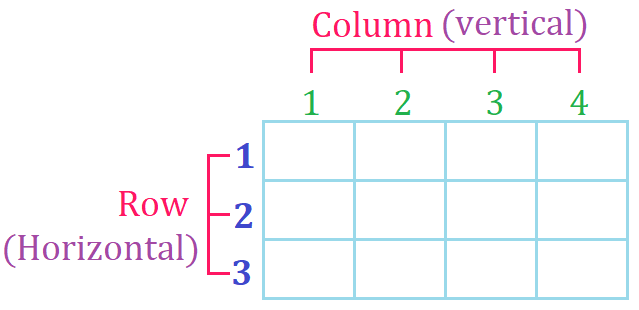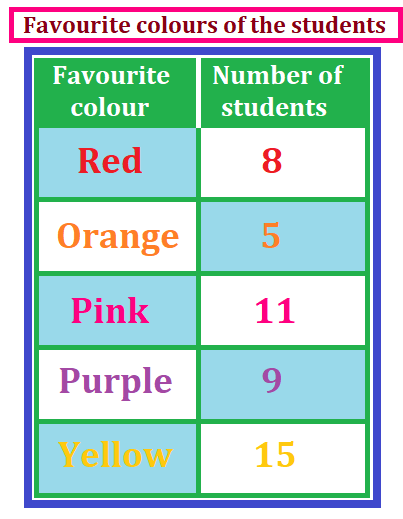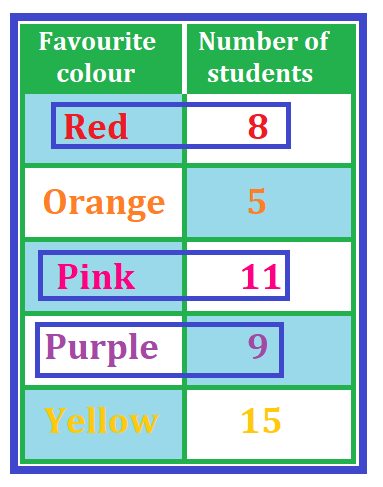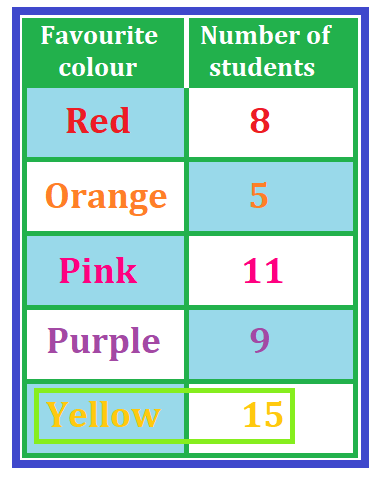# Math > Year 2

## Interpreting Tables

Table
A table is used to represent information clearly in rows and columns.

Parts

The title of the table tells us what the table is about.

The headings tell us what data is in each column and row.How to interpret the tables?

Let’s learn with the help of an example.

The following table shows the favourite colours of the students of year 1. Answer the following questions properly.We will observe the data given in the table carefully to answer the questions.

Question 1:

How many students like the orange colour?5 students like the orange colour.

Question 2:
What is the total number of students that like purple, pink and red colours?The word ‘total’ suggests that we need to add the numbers.

Number of students who like purple colour = 9

Number of students who like pink colour = 11

Number of students who like red colour = 8

Total = 9 + 11 + 8

= 28

Question 3:

Which colour is liked by 15 students?